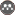Volume 24, Issue 2 (Summer 2020)                   JWSS 2020, 24(2): 55-68 | Back to browse issues page

BibTeX | RIS | EndNote | Medlars | ProCite | Reference Manager | RefWorks
Send citation to:Alizadeh A, Yaghoubi B, Shabanlou S. Simulation of Discharge Coefficient of Sharp-Crested Circular Weirs Using Hybrid ANFIS-Firefly Model. JWSS. 2020; 24 (2) :55-68
URL: http://jstnar.iut.ac.ir/article-1-3813-en.html
1. Department of Water Engineering, Kermanshah Branch, Islamic Azad University, Kermanshah, Iran. , aria_911ruf@yahoo.com
Abstract:   (147 Views)
In this study, the discharge coefficient of sharp-crested weirs located on circular channels was modeled using the ANFIS and ANFIS-Firefly (ANFIS-FA) algorithm. Also, the Monte Carlo simulations (MCs) were used to enhance the compatibilities of the soft computing models. However, the k-fold cross validation method (k=5) was used to validate the numerical models. According to the input parameters, four models of ANFIS and ANFIS-FA were introduced. Analyzing the numerical results showed that the superior model simulated the discharge coefficient as a function of the Froude number (Fr) and the ratio of flow depth over weir crest to the weir crest height) h/P(. The values of the mean absolute relative error (MARE), root mean square error (RMSE) and correlation coefficient (R) for the superior model were calculated 0.001, 0.002 and 0.999, respectively. However, the maximum error value for this study was less than 2%.